### Data evaluation of dynamic light scattering:

The central quantity of the evaluation of correlation spectroscopy is the decay time also named the correlation-time τ.  Unfortunately, it is not possible to detect this frequency with classical optical methods. As a consequence, it is substantial to use optical mixing techniques, like homogenous mixing, heterogenous mixing (homodyne and heterodyne techniques) and the self-beating method.

Self-beating spectroscopy:

The principle of self-beating spectroscopy is based on mixing of scattered light with the original light on analyzing the resulting signal with low-frequency intermediate signal. This is realized in three steps:

Consider a signal of ﬁeld strength E (R ,ω) with spectrum A , that has to be shifted into the lower frequencies. This happens with the aid of a local-oscillator signal. After this mixing procedure, it is possible to describe the scattered light with the  help of convolution integral of the incoming spectrum (Ap , spectrum of the photon ﬂux):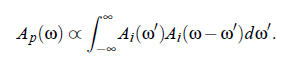On the one side, the photon ﬂux i(t ) is proportional to the light intensity,  |I (ω)| = |E (ω)|^2 .  On the other side, the considered Rayleigh line is of the shape of a Lorentz curve, with the the half-bandwidth ΓR and the center frequency ω_0: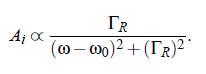For simple ﬂuids the half-bandwidth ΓR (see Fig.(3.16)) of the Lorenz-curve can be represented by: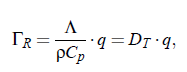here denotes D_T the thermal diffusivity, Λ the thermal conductivity, ρ the density,  C, the heat capacity at constant pressure p as well as q, the amount of the scattering vector. The next step is to ﬁnd a tool to measure the spectrum. This can be realized with the aid of Correlation Spectroscopy.

Correlation Spectroscopy: The Wiener-Khinchin theorem |Af (ω)|   = F Φ [f  (t )]  relates the signal f (t ) with the amount of the spectrum Af (ω). Here is F −1 the inverse  Fouriertransform  and Φ [f  (t )] is  the  autocorrelation  function  of f(t).  As mentioned before, the signal i(t) of the photon ﬂux is proportional to the light intensity I (t). Practically, in an experiment the autocorrelation function Φ [i], which is proportional to the autocorrelation function of Φ [I ], is determined.  Consequently, the following expression results: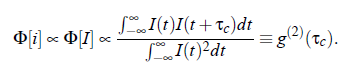In an experiment the interest is not focused on the intensity spectrum of the but in the spectrum of electric ﬁled E (t ).  Due to the well known relation I (t ) = |E (t )| , the expression (3.23) for the electric ﬁeld is given by: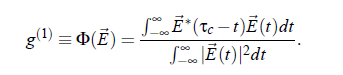Finally, the autocorrelation function g (2) of the intensity I (t ) and the autocorrelation function g (1) of the ﬁeld E (t) are related by the so-called Siegert relation: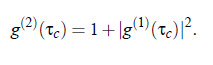In the DLS experiment one has to do with the Lorentz proﬁle. The autocorrelation function of this proﬁle is given by: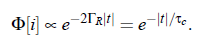This equation allows to determine the half-with  ΓR of  Rayleigh-line from the correlation-time 1/2ΓR  of the photon autocorrelation function.

Photon statistics: All considerations have been done with the assumption that i(t ) ∝  I (t ). In principle, this can be done for sufﬁciently high scattering count rates.  For  experiments  with  lower  rates  it  is  substantial  to  use  adequate statistics.